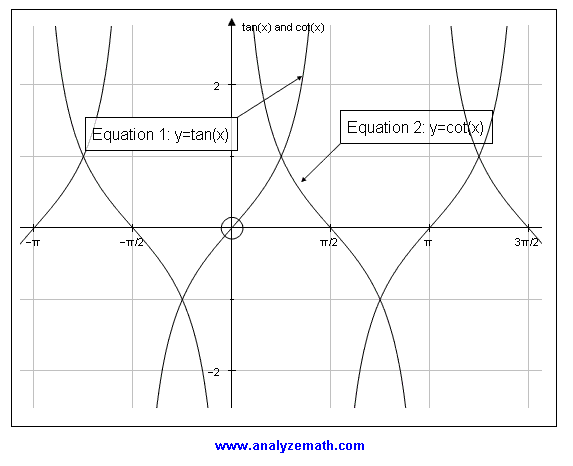# The Use of Graphing Software in the Mathematics Classroom (3)

 3.3 Explore the Graphs of Functions Task: Students use Autograph to graph the functions y = tan x and y = cot x and use graph transformations to find a relationship of the form tan(x) = a*cot(x + b) between the two graphs. They then use Autograph to check their answers graphically.Fig. 3: Explore relationship between tan(x) and cot(x). Students worked in pairs during this activity and were highly motivated. The whole activity was student centered in that students worked independently of the teacher and defined their own strategies to find a solution to the problem. Two important points to note about this activity. The question associated with this activity had many possible answers. Also the students were able to easily check their answers graphically. These two features of this activity made students more enthusiastic about using Autograph. 3.4 Explore Mathematical Problems and Solve them Graphically Problem: Find the length a and width b of a rectangle with a constant perimeter of 60 meters so that its area has a maximum value. Task: Students explore two possible representations of the problem and the link between them: a rectangle with constant perimeter and varying a and b, and a graph, in rectangular system, of the area of the rectangle as a function of a. Students approximate a and b that give an area maximum.Fig. 4: Rectangle with maximum area problem. This activity may be broadcast to all the students and therefore a whole class activity takes place. Students take part in this activity by answering open ended questions put by the instructor. Students are asked to find an answer to the problem graphically and in the second part of the activity, they are asked to explore an analytical solution to the problem. This activity helps students develop ideas in the field of optimization and also helps them find an analytical solution. 1, 2, 3, 4, 5,